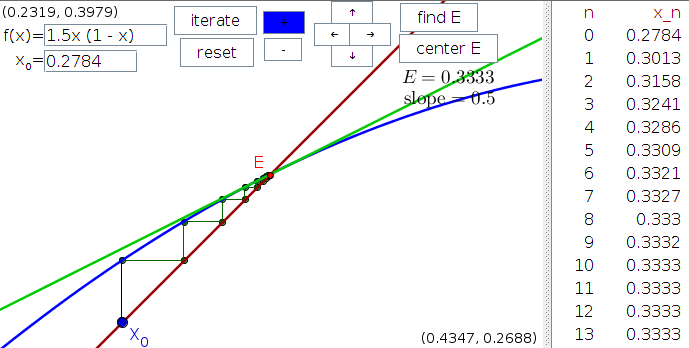# Math Insight

### Applet: Cobwebbing and linear approximations around equilibriaThe Java applet did not load, and the above is only a static image representing one view of the applet. The applet was created with Geogebra. The applet is not loading because it looks like you do not have Java installed. You can click here to get Java.Cobwebbing provides a way to visualize how a linear approximation to a function captures the behavior of its iteration near equilibria. Clicking the “iterate” button shows the behavior of the function iteration $x_n = f(x_{n-1})$ in a cobweb plot. The iterates $x_n$ are also displayed in the list at the right. To find an equilibrium (defined by $E=f(E)$), click the “find E” button, then click near a point where the graph of $f(x)$ (blue curve) intersects the diagonal $y=x$ (red line). If an equilibrium is found, the value of $E$ is displayed and a red point appears at the coordinates $(E,E)$. The linear approximation of $f$ around $E$ appears as a green line that is tangent to the graph of $f$ at the red point, and the value of its slope is displayed. If you click “center E,” the red point representing $E$ will be centered, and you can zoom in with the + button to see the behavior of $f$ right around $E$. What do you notice about the relationship of the linear approximation in green and the function in blue when you zoom in on the equilibrium? What does this say about the ability of the linear approximation to predict the behavior of $f$ around $E$?

You can change the function $f(x)$ by typing a new function in the box. You can change the initial point $x_0$ by typing a new value in the box or dragging the blue point. You can zoom in and out with the + and - buttons as well as pan in different directions with the buttons labeled by arrows.

Applet file: cobweb_linear_approximation_equilibrium.ggb